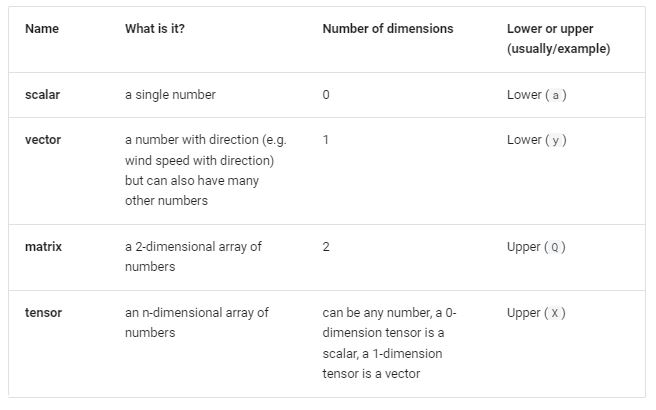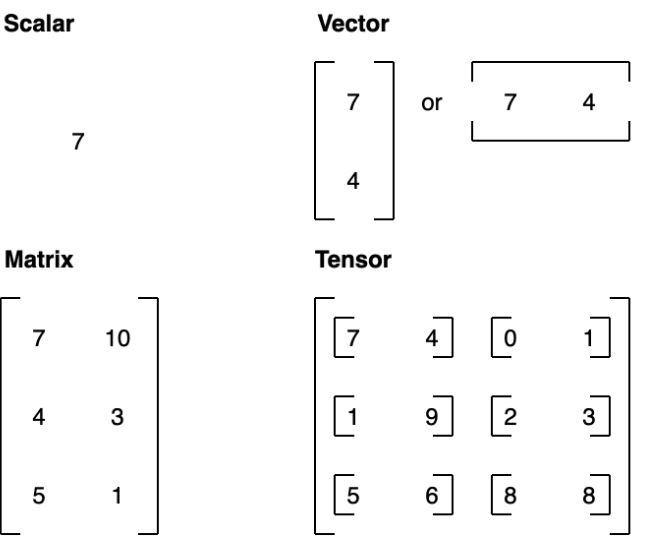# PyTorch deep learning framework

Rating & reviews (0 reviews)
Study notes

PyTorch is the most used deep learning framework on Papers With Code -Open-source machine learning and deep learning framework.
Allows you to manipulate and process data and write machine learning algorithms using Python code.
Used by: Meta (Facebook), Tesla and Microsoft as well as artificial intelligence research companies.

import torch
torch.__version__

Tesors
The fundamental building block of machine learning - represent data in a numericalway.Ascalaris a single number and in tensor-speak it's a zero dimension tensor.
Avectoris a single dimension tensor but can contain many numbers.
Matricesare as flexible asvectors, except they've got an extra dimension.
Tensor- multidimension array.Most common attributes you'll want to find out about tensors are:
• shape - what shape is the tensor? (some operations require specific shape rules)
• dtype - what datatype are the elements within the tensor stored in?
• device - what device is the tensor stored on? (usually GPU or CPU)
That's the debug point is something go wrong.
When the error messages show up, ask yourself:
• what shape are my tensors?
• whatdatatype are they
• whereare they stored?
float_32_tensor = torch.tensor([3.0, 6.0, 9.0],
dtype=None, # defaults to None, which is torch.float32 or whatever datatype is passed
device=None, # defaults to None, which uses the default tensor type
requires_grad=False) # if True, operations performed on the tensor are recorded
Result:
float_32_tensor.shape, float_32_tensor.dtype, float_32_tensor.device

Or better:
# Create a tensor
some_tensor = torch.rand(3, 4)

# Find out details about it
print(some_tensor)
print(f"Shape of tensor: {some_tensor.shape}")
print(f"Datatype of tensor: {some_tensor.dtype}")
print(f"Device tensor is stored on: {some_tensor.device}") # will default to CPU

Result:
tensor([[0.9672, 0.8122, 0.8128, 0.0442],
[0.1489, 0.9706, 0.7232, 0.9051],
[0.4688, 0.6139, 0.2822, 0.5096]])
Shape of tensor: torch.Size([3, 4])
Datatype of tensor: torch.float32
Device tensor is stored on: cpu

References:
PyTorch
00. PyTorch Fundamentals - Zero to Mastery Learn PyTorch for Deep Learning
mrdbourke/pytorch-deep-learning: Materials for the Learn PyTorch for Deep Learning: Zero to Mastery course. (github.com)

Tags: scalar / vector / matrix